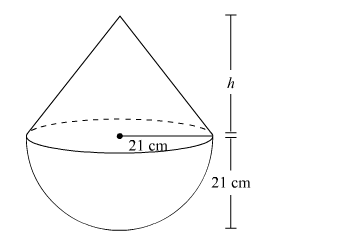# A toy is in the form of a hemisphere surmounted by a right circularQuestion:

A toy is in the form of a hemisphere surmounted by a right circular cone of the same base radius as that of the hemisphere. If the radius of the base of the cone is 21 cm and its volume is 2/3 of the volume of hemisphere, calculate the height of the cone and the surface area of the toy.

(Use $\pi=22 / 7$ ).

Solution:

Let the height of the conical part be h.

Radius of the cone = Radius of the hemisphere = r = 21 cm

The toy can be diagrammatically represented asVolume of the cone $=\frac{1}{3} \pi r^{2} h$

Volume of the hemisphere $=\frac{2}{3} \pi r^{3}$

According to given information:

Volume of the cone $=\frac{2}{3}$. Volume of the hemisphere

$\therefore \frac{1}{3} \pi r^{2} h=\frac{2}{3} \times \frac{2}{3} \pi r^{3}$

$\Rightarrow h=\frac{\frac{2}{3} \times \frac{2}{3} \pi r^{3}}{\frac{1}{3} \pi r^{2}}$

$\Rightarrow h=\frac{4}{3} r$

$\therefore h=\frac{4}{3} \times 21 \mathrm{~cm}=28 \mathrm{~cm}$

Thus, surface area of the toy = Curved surface area of cone + Curved surface area of hemisphere

$=\pi r l+2 \pi r^{2}$

$=\pi r \sqrt{h^{2}+r^{2}}+2 \pi r^{2}$

$=\pi r\left(\sqrt{h^{2}+r^{2}}+2 r\right)$

$=\frac{22}{7} \times 21 \mathrm{~cm}\left(\sqrt{(28 \mathrm{~cm})^{2}+(21 \mathrm{~cm})^{2}}+2 \times 21 \mathrm{~cm}\right)$

$=66(\sqrt{784+441}+42) \mathrm{cm}^{2}$

$=66(\sqrt{1225}+42) \mathrm{cm}^{2}$

$=66(35+42) \mathrm{cm}^{2}$

$=66 \times 77 \mathrm{~cm}^{2}$

$=5082 \mathrm{~cm}^{2}$